# 在 Power BI 中创建简单且图表友好的日期表``````
Classic Date =

VAR FirstYear = -- Customizes the first year to use

YEAR ( MIN ( Sales[Order Date] ) )

RETURN

FILTER (

CALENDARAUTO (),

YEAR ( [Date] ) >= FirstYear

),

"Year", YEAR ( [Date] ),

"Year Month", FORMAT ( [Date], "mmm yyyy" ),

"Year Month Number", YEAR ( [Date] ) * 12 + MONTH ( [Date] ),

"Month", FORMAT ( [Date], "mmm" ),

"Month Number", MONTH ( [Date] ),

"Day of Week", FORMAT ( [Date], "ddd" ),

"Day of Week Number", WEEKDAY ( [Date], 1 )

)``````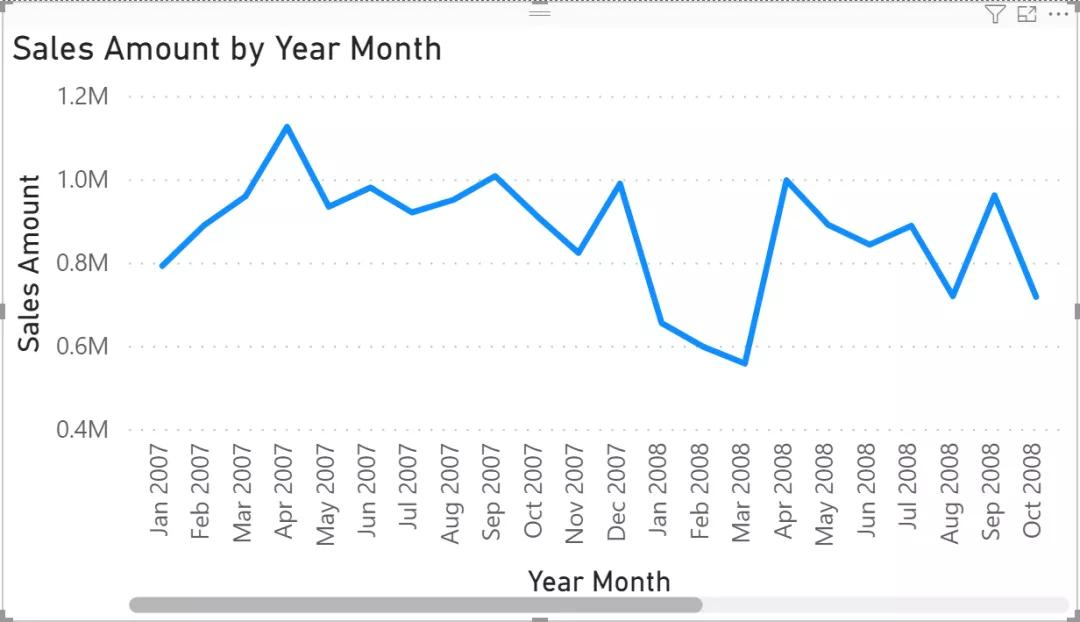``````Date =

VAR FirstYear = -- Customizes the first year to use

YEAR ( MIN ( Sales[Order Date] ) )

RETURN

FILTER (

CALENDARAUTO (),

YEAR ( [Date] ) >= FirstYear

),

"Year", DATE ( YEAR ( [Date] ), 12, 31 ),

"Year Month", EOMONTH ( [Date], 0 ),

"Month", FORMAT ( [Date], "mmm" ),

"Month Number", MONTH ( [Date] ),

"Day of Week", FORMAT ( [Date], "ddd" ),

"Day of Week Number", WEEKDAY ( [Date], 1 )

)``````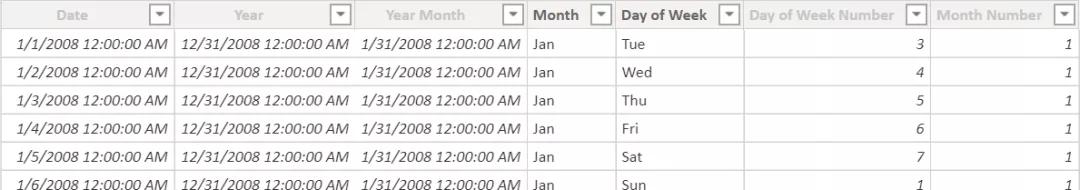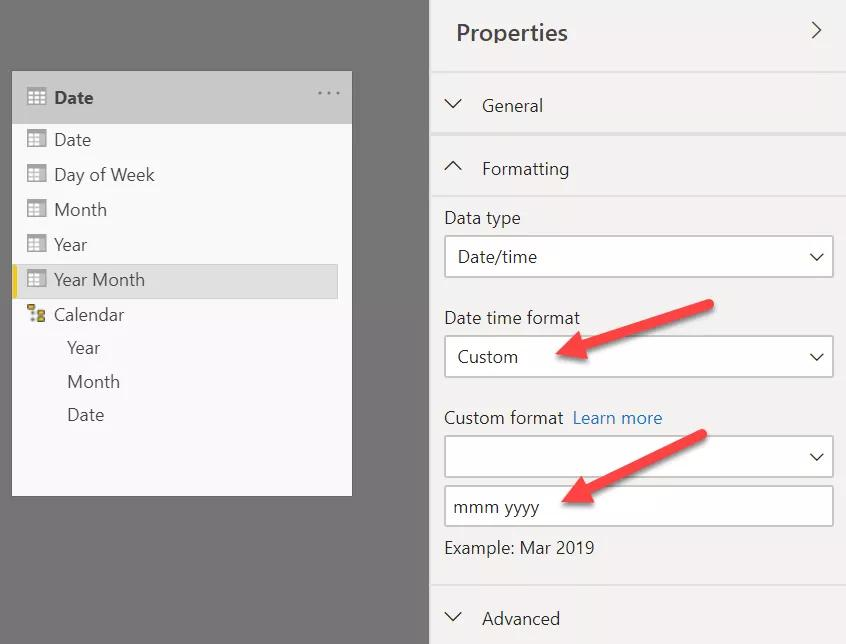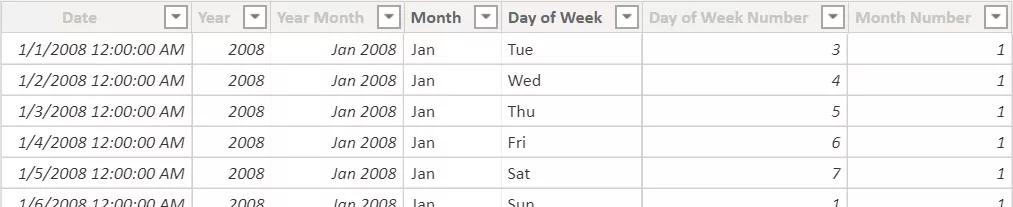Date表具有层次结构，即使这不会对格式产生副作用：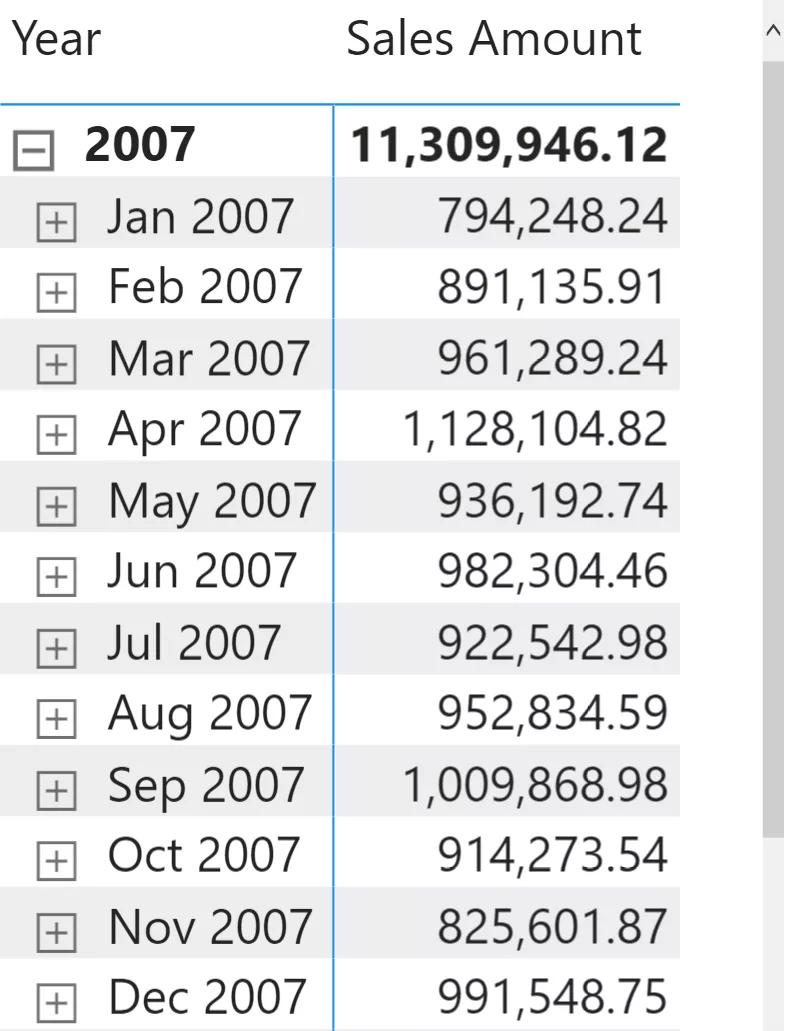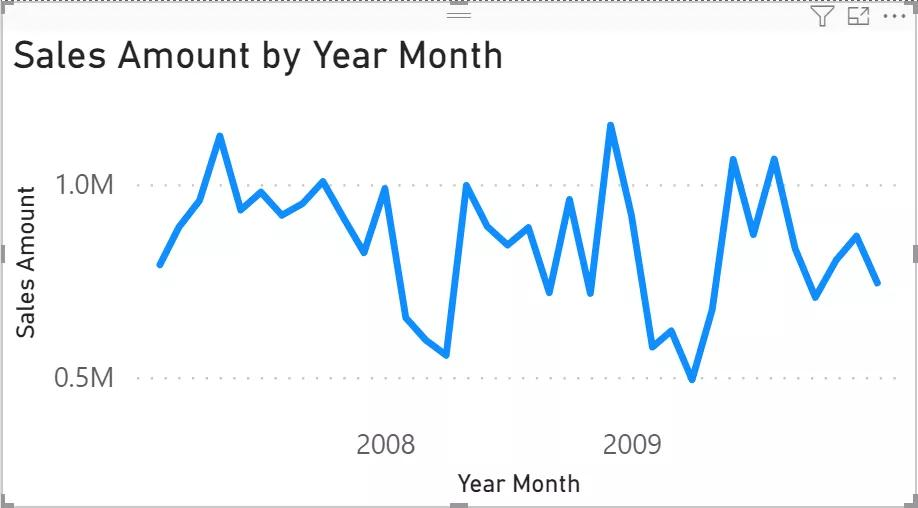• ## PowerPivot工坊原创文章，转载请注明出处！

如果您想深入学习微软Power BI，欢迎登录网易云课堂试听学习我们的“从Excel到Power BI数据分析可视化”系列课程。或者关注我们的公众号（PowerPivot工坊）后猛戳”在线学习”。Power Pivot工坊